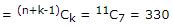Courses

# Test: Permutation And Combination- 2

## 20 Questions MCQ Test General Aptitude for GATE 2020 | Test: Permutation And Combination- 2

Description
This mock test of Test: Permutation And Combination- 2 for GMAT helps you for every GMAT entrance exam. This contains 20 Multiple Choice Questions for GMAT Test: Permutation And Combination- 2 (mcq) to study with solutions a complete question bank. The solved questions answers in this Test: Permutation And Combination- 2 quiz give you a good mix of easy questions and tough questions. GMAT students definitely take this Test: Permutation And Combination- 2 exercise for a better result in the exam. You can find other Test: Permutation And Combination- 2 extra questions, long questions & short questions for GMAT on EduRev as well by searching above.
QUESTION: 1

### A triangle is to be constructed in the xy-plane such that the x- and y- coordinates of each vertex are integers that satisfy the inequalities -3 ≤ x < 7 and 2 < y ≤ 7. If one of the sides is parallel to the x-axis, how many different triangles with these specifications can be constructed?

Solution:

Given: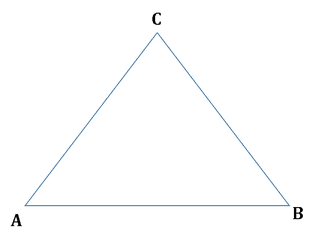• One side of the triangle is parallel to x – axis
• The x- and y – coordinates of each vertex are integers.
• For each vertex of the triangle,
• -3 ≤ x < 7
• 2 < y ≤ 7

To find: Number of different triangles that may be specified.

Approach:

1. The objective here consists of the following tasks:
• Task 1 – Choose the x-coordinates of the two vertices on the line parallel to x-axis
• Task 2 – Chose x-coordinate of the 3rd point
• Task 3 - Choose y-coordinate of the line parallel to x-axis
• Task 4 – Choose y-coordinate of the third point

Since each of these tasks must be performed in order to construct this triangle, the answer will be obtained by multiplying the number of ways of doing each task.

2. Using the given inequalities and the fact that only integer values of x and y are allowed, we’ll find the number of ways of doing each task.

Working Out:

• Choosing the x-coordinates of the 3 points
• Finding number of ways to do Task 1:
• -3 ≤ x < 7
• That is, -3 ≤ x ≤ 6
• Therefore, number of possible values of x is 10
• So, ways to choose x-coordinates of the 2 vertices on the line parallel to x-axis =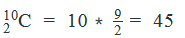• Finding number of ways to do Task 2
• Choices for third vertex’ x-coordinate = 10
• (If the x-coordinate of the third vertex is the same as the x-coordinates of either of the two vertices that form the line parallel to x-axis, we get a right triangle)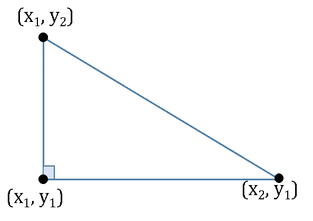• Choosing the y-coordinates of the 3 points
• Finding number of ways to do Task 3:
• 2 < y ≤ 3
• That is 3 ≤ y ≤ 7
• So, number of possible values of y is 5
• So, (Number of choices for y-coordinate of the line parallel to x-axis) = 5
• Finding number of ways to do Task 4:
• One of the 5 possible values of y is used up in Task 3
• So, (Number of choices available for third vertex’ y-coordinate) = 4
• So, total ways in which the triangle can be formed = 45*10*5*4 = 45*200  = 9000

Looking at the answer choices, we see that the correct answer is Option B

QUESTION: 2

### The players for a Tennis Mixed Doubles match are to be chosen from among 5 men and 4 women. In how many ways can the teams for the match be formed?

Solution:

Given:

• 5 Men
• 4 Women

To find: Number of ways to choose the 2 teams for a Tennis Mixed Doubles Match

Approach:

1. The Objective here consists of 3 Tasks:
• Task 1 – Select the 2 men who will play
• Task 2 – Select the 2 women who will play
• Task 3 – Arrange the 2 selected men and 2 selected women into 2 teams
2. Since all these 3 tasks need to be performed, Principle of Multiplication will be applicable.

So, Number of ways in which the players can be selected

= (Ways to Select 2 men out of 5)*(Ways to select 2 women out of 4)*(Ways to arrange the 2 selected men and 2 selected women into 2 teams)

Working Out:

• (Ways to Select 2 men out of 5) = 5C2
•
• (Ways to select 2 women out of 4) =4C2
•
• (Ways to arrange the 2 selected men and 2 selected women into 2 teams) = 2
• The number of ways to do Task 3 are 2 because in effect we have to select only 1 team – the remaining man and woman form the second team. So, if we fix M1, he can be paired either with W1 or with W2. The other woman is automatically teamed with M2. So, there are only 2 combinations possible.
• Therefore, Number of ways in which the players can be selected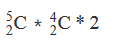• =10*6*2
• =120

Looking at the answer choices, we see that the correct answer is Option D

QUESTION: 3

### A soccer team of 11 players is to be formed out of a pool of 23 available players. The team should consist of 3 strikers, 4 midfielders, 3 defenders and 1 goalkeeper. The pool of 23 players consists of 6 strikers, 7 midfielders, 7 defenders and 3 goalkeepers. If Bruce, who is a striker, and David, who is a midfielder, should be a part of the team, what is the number of possible ways in which the team can be formed?

Solution:

Given

• Player available = 23
• Players to be selected = 11
• Strikers available = 6
• Strikers to be selected = 3
• Midfielders available = 7
• Midfielders to be selected = 4
• Defenders available = 7
• Defenders to be selected = 3
• Goalkeepers available = 3
• Goalkeepers to be selected = 1
• Striker Bruce and midfielder David have to be a part of the team

To Find: Number of ways of forming the team?

Approach

1. We need to find the number of ways in which a team of 11 players can be formed out of an available pool of 23 players.
1. The team should consist of certain number of strikers, midfielders, defenders and goalkeepers
2. The selection of the team can be divided into various tasks:
1. We need to select 3 strikers out of 6 available strikers. However, as we are told that Bruce has to be selected, we only need to select 2 more strikers out of 5 available strikers.
2. Taks-2: Selecting midfielders
1. We need to select 4 midfielders out of 7 available midfielders. However, as we are told that David has to be selected, we only need to select 3 more midfielders out of 6 available midfielders.
1. We need to select 3 defenders out of 7 available defenders.
1. We need to select 1 goalkeeper out of 3 available goalkeepers.

3.  As it’s an AND event,

Working Out

1. Number of ways to select 2 strikers out of 5 available strikers = 5C2 = 10
1. Number of ways to select 3 midfielders out of 6available strikers = 6C3 = 20
1. Number of ways to select 3 defenders out of 7 available defenders = 7C3 = 35
1. Number of ways to select 1 goalkeeper out of 3 available goalkeepers = 3C1 = 3
5. Total number of ways of forming the team = 10*20*35*3 = 21000

Thus, the team can be formed in 21000 ways.

QUESTION: 4

How many 3-letter words can be formed using the letters from the word GALE, if repetition is not allowed?

Solution:

Given: 4-letter word GALE

To find: Number of 3-letter words that can be formed without repeating any letters

Approach:

We will use the method of filling spaces to answer the question

Working Out:

1. Let’s draw the 3 spaces, to denote the 3-letter word we need to form: _ _ _
2. The objective of forming the word requires 3 tasks:
1. Task 1 – Fill Space 1
2. Task 2 – Fill Space 2
3. Task 3 – Fill Space 3
3. Since each of these 3 tasks need to be done to make the 3-letter word, we will multiply the number of ways to do each task:
4. Number of ways to fill Space 1 = 4 (G, A, L, E – all letters are available at this point)
5. Number of ways to fill Space 2 = 3
6. Number of ways to fill Space 3 = 2
7. So, (Number of 3-letter words) = 4*3*2 = 24

QUESTION: 5

How many 4 digit numbers greater than 4000 can be formed using the digits from 0 to 8 such that the number is divisible by 4?

Solution:

Given

• Digits to be used → 0 – 8
• Digits can be repeated
• Numbers to be divisible by 4

To Find: 4 digit integers > 4000 and divisible by 4?

Approach

1. We need to find the number of 4 digit integers greater than 4000 that are divisible by 4.
2. For an integer to be divisible by 4, the last two digits should be divisible by 4. So, we can divide this into the following tasks:
3. Task-1: Selecting the digit at thousands place
1. The thousands place can have a digit ≥ 4 and ≤ 8
4. Task-2: Selecting the digit at the hundreds place
1. The hundreds place can take any digit from 0-8
5. Task-3: Selecting the last 2 digits
1. So, we need to find the number of 2 digit integers that are divisible by 4 such that none of them contains a 9, i.e. we need to find the number of 2 digit integers divisible by 4 between 00 to 88, inclusive.
1. Please note that we can take 00 as one of the possibilities because the last 2 digits are a part of the 4 digit integer.
6. However, as we have taken 4 as one of the thousands place digit, there can be a case where the integer can be equal to 4000. So, we need to subtract this possibility when calculating our final answer.
7. So, number of 4 digit integers greater than 4000 = Task-1 * Task-2 * Task -3 – 1

Working Out

1. Task-1: Selecting the digit at thousands place
1. Number of ways to select the digit at thousands place = {4, 5, 6, 7, 8}, i.e. 5 ways..(1)
2. Task-2: Selecting the digit at hundreds place
1. Number of ways to select the digit at hundreds place = {0- 8) , i.e. 9 ways…..(2)
3. Task-3: Selecting the last 2 digits:
1. Number of multiples of 4 from 00 to 88, i.e. from 4*0 to 4*22 = 22 + 1 = 23
4. Number of 4 digit integers greater than 4000 = 5 * 9 * 23 – 1 = 1035 – 1 = 1034

QUESTION: 6

A company interviewed 5 applicants each for the posts of the Director and the  President. If Jack and Jill were the only applicants who were interviewed for both the posts and an applicant can be selected for only one of the posts, what is the number of ways in which the company can select its Director and President from the interviewed applicants?

Solution:

Given

• Number of applicants for the post of Director = 5
• Number of applicants for the post of President = 5
• Jack and Jill are interviewed for both the posts
• Hence, total number of applicants = 5 + 5 – 2 = 8

To Find: Number of ways to select the Director and the President?

Approach

1. We need to find the number of ways in which a company can select its Director and President given that Jack and Jill were the only two applicants who were interviewed for both the posts.
2. As Jack and Jill were interviewed for both the positions, following cases are possible:
3. Case-I: Neither of Jack or Jill are selected for any of the posts
1. In this case we are left with 3 applicants each for the post of director and the president. We need to select 1 applicant each from the pool of 3 applicants each for the post of director and the president
4. Case-II: Only one of Jack or Jill is selected. Following cases are possible:
1.  Either of Jack or Jill is selected for the post of director:  So, there are 2 ways to select the director and for the post of president we need to select 1 applicant from the remaining 3 applicants.
2. Either of Jack or Jill is selected for the post of president:  So, there are 2 ways to select the president and for the post of director we need to select 1 applicant from the remaining 3 applicants.
5. Case-III: Both Jack and Jill are selected
1. In this case, Jack can be selected for the post of the director or the president and Jill can be selected for the other post.
6. Total ways = Possible ways in case-I + Possible ways in case-II + Possible ways in case-III

Working Out

1. Case-I: Neither of Jack or Jill are selected for any of the posts
1. Number of ways to select the director = Number of ways to select 1 applicant from the remaining 3 applicants =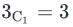• Number of ways to select the president = Number of ways to select 1 applicant from the remaining 3 applicants =• Total number of ways = 3 * 3 = 9…………(1)
• Case-II: Only one of Jack or Jill is selected
1. Either of Jack or Jill is selected for the post of the director
1. Number of ways to select a director = 2
2. Number of ways to select the president = Number of ways to select 1 applicant from the remaining 3 applicants =• So, number of ways = 3 * 2 = 6
• Either of Jack or Jill is selected for the post of the president
1. Number of ways to select the president = 2
2. Number of ways to select the director= Number of ways to select 1 applicant from the remaining 3 applicants =So, number of ways = 3 * 2 = 6
1. Total number of ways = 6 + 6 = 12……..(2)
2. Case-III: Both Jack and Jill are selected
1. So, Jack can be selected for the post of the president or the director and Jill will be selected for the other post
2. Number of ways = 2……..(3)
3. Using (1), (2) and (3), we have
1. Total ways to select the director and the president = 9 + 12 + 2 = 23

Hence, there are 23 ways in which the company can select its director and the president.

QUESTION: 7

How many positive 4-digit integers are divisible by 20 if the repetition of digits is not allowed?

Solution:

Given:

• Positive 4 – digit numbers
• Repetition is not allowed

To find: Number of positive 4-digit numbers that are divisible by 20

Approach:

1. A number is divisible by 20 if its tens and units digits are: {0 0 or 2 0 or 4 0 or 6 0 or 8 0 } respectively
• Since repetition of digits is not allowed, the case of 0 0 can be ruled out.
2. Thus, there are 4 possible combinations of tens and units digits for which a 4-digit number can be divisible by 20.
3. So, to answer the question, we will find out, how many 4-digit numbers will have a particular combination of tens and units digits.
• That is, how many 4-digit numbers will have their tens and units digits as:
• 2 0?
• 4 0 ? and so on.

Working Out:

• Let’s first find out how many 4-digit numbers will have their tens and units digits as 2 0
• Out of the 10 available digits ( 0 – 9, inclusive), two (0 and 2) have already been used up
• So, number of digits available for the hundreds digit = 10 – 2 = 8
• It is important to remember that repetition is not allowed in this question.
• And, the number of digits available for the thousands digit = 7
• So, total 4- digit numbers whose tens and units digits are 2 0 = 8*7 = 56
• The calculations will be similar for the other combinations of tens and units digits as well. And the total 4-digit numbers that have each of those combinations will also be 56
• So, the total number of 4 – digit numbers whose tens and units digits are {2 0 or 4 0 or 6 0 or 8 0} = 56 + 56 + 56 + 56 = 224
• Therefore, Number of positive 4-digit numbers that are divisible by 20 = 224

Looking at the answer choices, we see that the correct answer is Option C

QUESTION: 8

A salesman has five chocolates each of three different varieties. If he has to sell 9 chocolates to 9 different people and he can sell at most two varieties of chocolates, in how many different ways can he sell the chocolates?

Solution:

Given

• Total chocolates = 5*3 = 15
• Chocolate of variety A = 5
• Chocolate of variety B = 5
• Chocolate of variety C = 5
• Number of chocolates to be sold = 9
• Number of people to whom chocolates are to be sold = 9
• The shopkeeper can sell at-most 2 varieties of chocolates

To Find: Number of ways in which the chocolates can be sold?

Approach

1. We need to find the number of ways in which the shopkeeper can sell 9 chocolates to 9 different people such that at-most only 2 varieties of chocolates are sold.
1. So, the shopkeeper can sell either 1 variety of chocolate or 2 varieties of chocolates. As none of the varieties have 9 or more chocolates, he will have to sell exactly 2 varieties of chocolates
2. Task-1: Selecting the varieties of chocolates
1. We need to select 2 varieties of chocolates from 3 varieties of chocolates
3. Task-2: Selecting the variety for which all the chocolates will be distributed
1. Total number of chocolates in 2 varieties = 10
2. Number of chocolates that the shopkeeper needs to distribute = 9
3. As the 2 varieties of chocolates have in a total of 10 chocolates and the shopkeeper needs to distribute 9 chocolates, we will need to select 1 variety of chocolate out of the 2 selected varieties of chocolates for which all the 5 chocolates will be distributed
4. Task-3: Distributing 9 chocolates among 9 different people
1. We need to find the number of ways in which 9 chocolates can be distributed among 9 different people.
2. However, we should take into account the fact that out of the 9 chocolates, 5 chocolates are of one variety and 4 chocolates are of the other variety, i.e. they are identical.
3. In effect, we need to distribute 9 objects, in which 5 objects are identical and the rest 4 objects are identical.
5. Possible number of ways in which the shopkeeper can distribute the chocolates = Task-1 * Task-2 * Task-3

Working Out

1. Task-1: Selecting the varieties of chocolates
1. Number of ways to select 2 variety of chocolates out of the 3 varieties = 3C2 = 3 .....(1)
• Task-2: Selecting the variety for which all the chocolates will be distributed
1. Selecting 1 variety out of the 2 selected varieties =2C1 = 2 …….(2)
• Task-3: Distributing 9 chocolates among 9 different people
1. Number of ways to distribute 9 chocolates among 9 different people such that 5 chocolates are identical and the rest 4 chocolates are identical =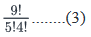Combining (1), (2), (3), we have
2. Possible number of ways in which the shopkeeper can distribute the chocolates =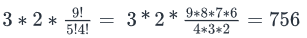3. Thus, there are 756 ways in which the shopkeeper can distribute the chocolates.

QUESTION: 9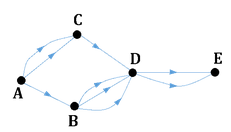The figure above shows the different alternate routes possible to go from point A to point E. The arrows show the allowed direction of motion on each route. What is the total number of ways to go from point A to E?

Solution:

Given: A figure with multiple routes to go from A to E

To find: Total number of ways to go from A to E

Approach:

1. There are 2 routes to go from A to E – ACDE or ABDE. Since you can take only one of these routes at one time, we will add the number of ways to go down each route

So, (Number of ways to go from A to E) = (Number of ways to go down the route ACDE) + (Number of ways to go down the route ABDE)

2. So, to answer the question, we need to work out the number of ways to go down each route

Working Out:

• Number of ways to go down the route ACDE
• To go down the route ACDE, you need to do the following tasks:
• Task 1 – Go from A to C
• AND Task 2 – Go from C to D
• AND Task 3 – Go from D to E
• Since each of these 3 tasks need to be done, the number of ways to do each task will be multiplied
• So, (Number of ways to go down the route ACDE) = (Number of ways to go from A to C)*(Number of ways to go from C to D)*(Number of ways to go from D to E)
• =2*1*2
• = 4
• Number of ways to go down the route ABDE
• To go down the route ABDE, you need to do the following tasks:
• Task 1 – Go from A to B
• AND Task 2 – Go from B to D
• AND Task 3 – Go from D to E
• Since each of these 3 tasks need to be done, the number of ways to do each task will be multiplied
• So, (Number of ways to go down the route ABDE) = (Number of ways to go from A to B)*(Number of ways to go from B to D)*(Number of ways to go from D to E)
• =1*3*2
• = 6
• (Number of ways to go from A to E) = 4 + 6 = 10

QUESTION: 10

The editor of an anthology of short stories has to select authors from a pool of 12 authors, of which 4 are female. If the anthology is to include the work of 8 authors of which at least 2 must be female, in how many ways can the editor select the authors?

Solution:

Given:

• Total 12 Authors
• Female (F) = 4
• So, Male (M) = 12 – 4 = 8
• 8 authors to be selected, at least 2 must be F

To find: Number of ways in which this selection may be made

Approach:

1. (Number of ways to select 8 authors with at least 2 F) = (Ways to select 2F and 6M) + (Ways to select 3F and 5M) + (Ways to select 4F and 4M)
1. In any of these 3 possible ways to fulfil the condition of choosing at least 2 F, we need to select F and we need to select M
2. So, (Ways to select 2F and 6M) = (Ways to select 2F out of 4F)*(Ways to select 6M out of 8M)
3. We can write similar equations corresponding to the other 2 ways as well.

Working Out:

• (Number of ways to select 8 authors with at least 2 F) =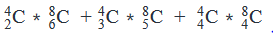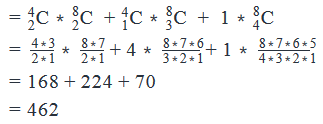Looking at the answer choices, we see that the correct answer is Option D

QUESTION: 11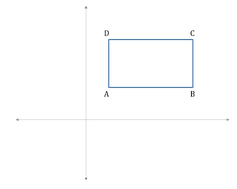The figure above shows a rectangle ABCD in the xy- coordinate plane. The sides AB and AD are parallel to the x- and the y- axis respectively. How many squares of side 1 unit that lie on or inside the rectangle ABCD can be drawn?

(1) The length of side AB is 6 units

(2) The coordinates of points A and C are (3,2) and (9, 5)

Solution:

Steps 1 & 2: Understand Question and Draw Inferences

Given: Rectangle ABCD in which AB is parallel to the x-axis and AD is parallel to the y-axis

To find: Number of squares of side 1 unit that can be drawn inside or on the rectangle ABCD

• To understand how to solve this question, let’s draw one square APQR, in which AP = PQ = 1 unit, in the rectangle ABCD: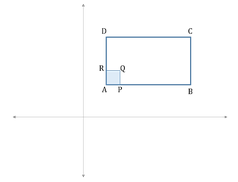Let’s find the Number of squares of side 1 unit that can be formed in the same row as square APQR (let’s call this number r):

• If AB is an integer, then r =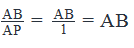• If AB is not an integer, then r = the greatest integer less than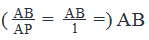• For example, say AB = 6.5
• The number of squares of length 1 unit that can be formed in 1 row will be 6 in this example (because how do you draw 6.5 squares? You cannot.)
• The figure below shows a sample illustration of how squares can be formed in the same row as square APQR. We can continue forming the squares in the same row till we reach side BC. The total number of squares in this row, as we saw above, will be AB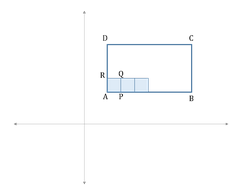Similarly, we can find that the Number of squares that can be formed in the same column as square APQR (let’s call this number c) =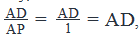• The greatest integer less than AD if AD is not an integer

(Total number of squares that can be formed in the rectangular region ABCD) = r*c

Since the values of r and c depend on AB and AD, we need to find the values of AB and AD, that is, the length and breadth of rectangle ABCD.

Step 3: Analyze Statement 1 independently

Statement 1 says that ‘The length of side AB is 6 units’

• This statement doesn’t give us the measure of AD
• Therefore, it is not sufficient to answer the question

Step 4: Analyze Statement 2 independently

Statement 2 says that ‘The coordinates of points A and C are (3,2) and (9, 5)’

• Remember that AB (and therefore CD) is parallel to x- axis.
• Therefore, (y-coordinate of A) = (y-coordinate of B) = 2
• And, (y-coordinate of D) = (y-coordinate of C) = 5

• Since AD (and therefore BC) is parallel to y – axis:
• (x-coordinate of A) = (x-coordinate of D) = 3
• And, (x-coordinate of B) = (x-coordinate of C) = 9

• Therefore, the coordinates of all points of the rectangle can be represented visually as below: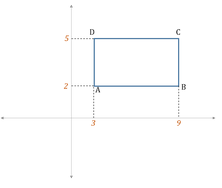• So, AB = 9 – 3 = 6
• And, AD = 5 – 2 = 3
• Since we now know the values of AB and AD, we can calculate r and c, and hence can get to the answer
• So, Statement 2 alone is sufficient to answer the question

Step 5: Analyze Both Statements Together (if needed)

Since we’ve already arrived at a unique answer in Step 4, this step is not required

QUESTION: 12

The lowest integer that has both positive integers x and y as its factors can be written in the form of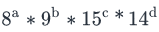If x can be written as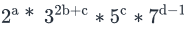, where a, b, c and d are positive integers, what are the possible number of values that y can take ?

Solution:
• a, b, c, d are integers > 0
• LCM (x, y) =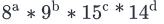LCM(x, y) =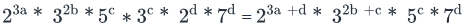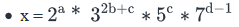To Find: Number of values y can take?

Approach

1. We are given the LCM (x, y) and the value of x. We know that LCM (x, y) will take the highest powers of the prime factors present in x and y.
2. So, if the LCM (x, y) contains a power of a prime factor greater than that in x, that power of that prime factor must be present in y.
3. Also, if the LCM (x, y) contains a power of a prime factor equal to that in x, y can take any power of that prime factor not greater than the power of the prime factor present in the LCM (x, y)
1. For example: If LCM(x, y) = 25 *  3and x =25 *32  , then y can take any power of the prime factor 2, not greater than 5. So, y can have either of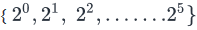, but it can take only one value of prime factor 3, which is 34

4. So, number of possible values of y = Number of ways in which 2 can be present in y * Number of ways in which 3 can be present in y * Number of ways in which 5 can be present in y* Number of ways in which 7 can be present in y

Working Out

1. LCM ( x, y) =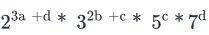and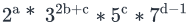2. Prime factors for which their powers in LCM (x, y) is greater than that in x

and 7 do not have the same powers in LCM (x, y) and x

1. 2 can be present in only one form in y = 23a+d .............(1)
2. 7 can be present in only one form in y = 7d…………….(2)

3. Prime factors for which their powers in LCM (x, y) and x are same

a. 3 and 5 have the same powers in both LCM (x, y) and x

1. Hence, possible number of ways in which 3 can be present in y =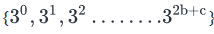, i.e

a total of (2b+c+1) ways………(3)

2. Possible number of ways in which 5 can be present in y =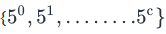, i.e. a total of (c+1) ways…….(4)

4. So, number of possible values of y = 1 * 1 * (2b+c+1) * (c+1) = (2b+c+1) (c+1)

So, there can be (2b+c+1) (c+1) values of y.

QUESTION: 13

A code is formed by combining one of the letters from A- Z and two distinct digits from 0 to 9 such that if the letter in the code is a vowel, the sum of the digits in the code should be even and if the letter in the code is a consonant, the sum of the digits in the code should be odd. If the code is case-sensitive (for example, A is considered to be different from a), how many different codes are possible?

Solution:

Given

• Code consists of 1 alphabet from a-z and 2 distinct digit from 0-9
• If the letter is vowelà sum of the digits should be even
• If the letter is a consonantà sum of the digits should be odd
• Letter is case sensitive

To Find: Number of possible codes?

Approach

1. We need to find the number of possible codes with 1 letter and 2 distinct digits such that if the letter is a vowel, the sum of the digits is even and if the letter is a consonant, the sum of the digits is odd
1. Also, the letter is case sensitive
2. Following cases are possible:
3. Case-I: If the letter selected is a vowel
1. We need to select 1 vowel out of 5 vowels. We should also take into account that the vowel can be in either small case or large case
2. The sum of the digits should be even. This is possible in the following cases:
1. Both the digits are even→ So, we need to select 2 even digits from a set of 5 even digits (0, 2, 4, 6 or 8)
2. Both the digits are odd→ We need to select 2 odd digits from a set of 5 odd digits(1, 3, 5, 7 or 9)
3. Lastly we should arrange the 1 letter and 2 digits selected
4. Total number of codes formed in case-I = Number of ways to select a vowel  * Number of ways in which sum of two digits can be even * Number of ways to arrange 1 letter and 2 digits

4.  Case-II: If the letter selected is a consonant

1. We need to select 1 consonant out of 21 consonants. We should also take into account that the consonant can be in either small case or large case
2. The sum of the two digits should be odd. This is possible if one of the digit is odd and the other is even.
3. So, we need to select 1 even digit out of 5 even digits (0, 2, 4, 6 or 8) and 1 odd digit out of 5 odd digits (1, 3, 5, 7, or 9)
4. Total number of codes formed in case-II = Number of ways to select a consonant * Number of ways in which sum of two digits can be odd * Number of ways to arrange 1 letter and 2 digits

5.  Total number of ways of forming a code = Number of codes formed in Case-I + Number of codes formed in Case-II

Working Out

1. Case-I: If the letter is a vowel
1. Number of ways to select a vowel = 5 C= 5
2. Both the digits are even→ Number of ways to select 2 even digits out of 5 even digits =  5C= 10
3. Both the digits are odd→ Number of ways to select 2 odd digits out of 5 odd digits = 5C= 10
4. Number of ways in which sum of two digits can be even = 10 + 10 = 20
5. Number of ways in which 1 letter and 2 digits can be arranged = 3!
6. Number of codes formed in case-I= 5 * 2 * 20 * 3! = 1200……..(1)

Case-II: If the letter is a consonant

1. Number of ways to select a consonant =  21C1 =21
2. Number of ways to select 1 odd digit from 5 odd digits and 1 even digit from 5 even digits =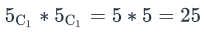Number of ways in which 1 letter and 2 digits can be arranged = 3!

1. Number of codes formed in case-II: 21 *2 * 25 *3! = 6300……..(2)
2. Total number of codes formed = 1200 + 6300 = 7500
3. Hence, there are 7500 codes possible.

QUESTION: 14

Micky has 10 different letters and 5 different envelopes with him. If he can only put one letter in an envelope, in how many ways can the letters be put in the envelopes?

Solution:

Given

• Number of letters = 10
• Number of envelopes = 5

To Find: Number of ways in which 5 letters can be put in 5 envelopes?

Approach

1. We need to find the number of ways in which 5 different letters out of 10 available letters can be put in 5 different envelopes.
2. Task-1: Selecting 5 letters out of 10 letters
1. We need to select 5 letters out of 10 available letters that are to be put in the envelopes
3. Task-2: Putting 5 different letters in 5 different envelopes
1. We need to distribute 5 different letters in 5 different envelopes

Working Out

1. Task-1: Selecting 5 letters out of 10 letters
1. Number of ways to select 5 different letters out of 10 different letters = 10 C5……(1)
• Task-2: Putting 5 selected letters in 5 different envelopes
1. 1st letter has an option of being put in 5 envelopes
2. 2nd letter has an option of being put in any of the remaining 4 envelopes
3. …….
4. ….
5. 5th letter has an option of being put in only 1 remaining envelope.
6. So, total ways in which 5 different letters can be put in 5 different envelopes = 5 *4*3*2*1 = 5!........(2)
• Combining (1) and (2), we have
1. Number of ways of putting 5 different letters out of 10 different letters in 5 different envelopes = 10C5 * 5!
QUESTION: 15

In how many ways can 12 different books be distributed equally among 4 different boxes?

Solution:

Given:

• Not applicable

To find: Number of ways to distribute 12 different books equally among 4 different boxes.

Approach:

1. The Event here consists of 2 tasks:
• Task 1 – Sort the 12 books into 4 stacks of 3 books each
• Task 2 – Put the 4 stacks in the 4 boxes
• Since both these tasks need to be done, we will multiply the number of ways of doing them.
• So, Number of ways in which the Event can happen = (Number of ways to do Task 1)*(Number of ways to do Task 2)

Working Out:

• Finding Number of ways to do Task 1
• Let’s first see in how many ways can the first stack be created.
• The first stack, like the other 3 stacks, will have 3 books in it.
• We have 12 books in total
• So, the number of ways in which we can choose the 3 books (out of the 12 books) that will go into first stack = 12C3
• Now, let’s consider the creation of the 2nd stack
• At this point, 9 books are left (since 3 have already gone into the first stack)
• So, the number of ways in which we can choose the 3 books that will go into the second stack = 9C3
• Similarly, the number of ways in which the 3 books for the 3rd stack can be chosen = 6C3
• And, finally, the number of ways in which the 3 books for the last stack can be chosen = 3C3
• Now, Task 1 involves the creation of Stack 1 and Stack 2 and Stack 3 and Stack 4
• So, Number of ways to do Task 1 =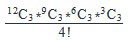• (We are dividing by 4! Since the order of choosing stacks doesn’t matter)

• Finding Number of ways to do Task 2
• Now that we have the 4 stacks containing 3 books each ready from Task 1, our job in Task 2 is to put these 4 stacks in the 4 boxes.
• So, essentially, we have to arrange the 4 stacks in 4 spaces (each space denotes a box)
• The number of ways in which this can be done = 4!

• Finding the Number of ways in which the Event can happen
• So, Number of ways in which the Event can happen =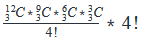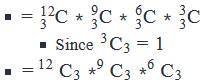Looking at the answer choices, we see that the correct answer is Option C

QUESTION: 16

The host of a television debate show has to select a 4-member discussion panel out of a list of 22 willing candidates that includes 5 politicians and 6 businessmen. If the list includes candidates from at least 4 professions and no two members of the discussion panel are to be of the same profession, then in how many ways can the panel be constituted?

(1)  The list includes 5 journalists and 2 authors

(2)  The list includes only 1 profession from which there are fewer than 3 candidatesStatement (1) ALONE is sufficient, but statement (2) alone is not sufficient to answer the question asked.

Solution:

Steps 1 & 2: Understand Question and Draw Inferences

Given:

• Total number of candidates on the list = 22
• Let number of politicians and businessmen be P and B respectively
• P = 5
• B = 6
• So, candidates in other professions = 22 – 5 – 6 = 11

• Total number of professions in the list is greater than or equal to 4
• This means, there are at least 2 other professions in the list
• The maximum number of other professions can be 11 (this will happen in the case where each of the 11 candidates in other professions has a distinct profession)
• So, the number of other professions lies between 2 and 11, inclusive

To find: Number of ways to constitute a 4 – member panel, such that all members have different professions

• If the total number of professions = 4
• This means, there are 2 other professions. Let’s label these as P1 and P2
• So, the distribution of the 22 candidates among these 4 professions will be as under:
• Politics = 5
• P1 = x (assuming this number)
• P2 = 11 – x
• So, the tasks involved in fulfilling the objective will be:
• Task 1 – Select 1 out of 5 politicians AND
• Task 3 – Select 1 out of x P1 people AND
• Task 4 – Select 1 out of 11 – x P2 people
• So, the objective equation will be:

(RequiredNumberofways)=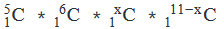• Thus, to answer this question, we need to know the value of x
• If the total number of professions > 4
• So, the total number of professions is 5 or more
• This means, there are 3 or more other professions. Let’s label these as P1, P2, P3 etc.
• So, the distribution of the 22 candidates among these 4 professions will be as under:
• Politics = 5
• P1 = x (assuming this number)
• P2 = y (assuming this number)
• P3 = z (assuming this number)
• And so on, where x + y + z + .  . . = 11

• So, here the objective consists of 2 events:
• Event 1 - Determine which 4 professions should be represented on the panel
• If the total number of professions is N, then the number of ways in which Event 1 can happen = N4C
• Depends on the value of N

• Event 2 - Determine the number of ways in which candidates from each of the 4 chosen professions can be represented. The computation for this event will be done using the same process as shown above in the case where the number of professions was 4
• This calculation will depend on the number of candidates in each profession

• So, in this case, we need to know the number of professions and the number of candidates in each profession

• Thus, we observe that in order to answer the question, we need to know:

• the number of professions and
• the number of candidates in each profession

Step 3: Analyze Statement 1 independently

• The list includes 5 journalists and 2 authors
• Let number of journalists and authors be J and A respectively
• J = 5
• A = 6
• So, candidates in still unknown professions (apart from Business, Politics, Journalism and Authorship) = 11- 5 - 2 = 4
• There may be 1 unknown profession with 4 candidates in it (so, total number of professions = 5)
• Or, there may be 2 unknown professions, with 2 candidates each in it (thus, total number of professions = 6)
• Or, 3 unknown professions with 1, 1 and 2 candidates in each (thus, total number of professions = 7)
• Or 4 unknown professions with 1 candidate each (thus, total number of professions = 8)

So, since we have not been able to determine a unique number of professions, Statement 1 alone is not sufficient.

Step 4: Analyze Statement 2 independently

• The list includes only 1 profession from which there are fewer than 3 candidates
• Politics has 5 candidates
• This means, the profession mentioned in Statement 2 must be one of the ‘other professions’
• This particular profession, let’s call it P1 has either 1 or 2 candidates
• We’ve already calculated in Steps 1 and 2 that Total candidates in ‘other professions’ = 11
• So, the number of candidates in professions apart from Politics, Business or P1 (let’s call these ‘unknown professions’) is 11 – 1 or 11 – 2, that is, 10 or 9
• There may be 1 unknown profession with 9 or 10 people in it (thus, total number of professions = 4)
• Or, there may be 2 unknown professions (thus total number of professions = 5) and the 9 or 10 people may be split between these 2 unknown professions in many possible ways: (1,8) or (2, 7) etc.
• And so on. .  .

We quickly see that multiple values for the number of professions and for the number of people in each profession are possible here.

So, Statement 2 is not sufficient to answer the question

Step 5: Analyze Both Statements Together (if needed)

• From Statement 1:
• Number of candidates in still unknown professions = 4
• Possible split of these 4 candidates among distinct unknown professions = {4, 0}, {3, 1}, {2, 2}, {2, 1, 1}, {1, 1, 1, 1}
• From Statement 2:
• There is only one profession with less than 3 candidates
• Combining the two statements:
• The only split of the 4 candidates among distinct unknown professions = {4, 0}
• Thus, the split of the 22 candidates among different professions is as under:
• Politics = 5
• Journalism = 5
• Authorship = 2
• Unknown Profession 1 = 4

Since we now know the number of professions and the number of candidates in each profession, we will be able to answer the Q.

The 2 statements together are sufficient to answer the question.

QUESTION: 17

What is the value of even positive integer n?

(1) The number of ways to choose 2 items out of n distinct items is 28

(2) The number of ways to choose 2 prime numbers out of the first n/2  positive integers is 1

Solution:

Steps 1 & 2: Understand Question and Draw Inferences

Given: Even Integer n > 0

To find:  n = ?

Step 3: Analyze Statement 1 independently

Statement 1 says that ‘The number of ways to choose 2 items out of n distinct items is 28’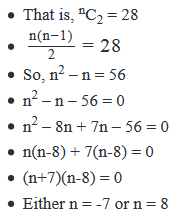• Rejecting the negative value since n is given to be a positive integer
• Therefore, n = 8
• Since Statement 1 gives a unique value of n, it is sufficient

Step 4: Analyze Statement 2 independently

Statement 2 says that ‘The number of ways to choose 2 prime numbers out of the first n/2

positive integers is 1’

• Let there be p prime numbers in the first n/2 positive integers
• So, pC2 = 1
• This equation is satisfied by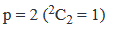• So, there are 2 prime numbers in the first n2
•  positive integers
• These will be the 1st 2 prime numbers: {2, 3}
• Since 2 and 3 feature in the first n/2 positive integers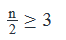• That is, n≥6
• However, since 5 doesn’t feature in the first n2
•  positive integers (otherwise the total number of prime numbers in the n2 positive integers would have become three – 2, 3 and 5), n/2<5
• That is, n < 10

• So, 10>n>6
• The even numbers that lie in this range are: {6, 8}
• Therefore, there are 2 possible values of n
• Statement 2 is not sufficient to lead us to a unique value of n

Step 5: Analyze Both Statements Together (if needed)

Since we’ve already arrived at a unique answer in Step 3, this step is not required

QUESTION: 18

In how many ways can 10 software engineers and 10 civil engineers be seated in a round table so that they are positioned alternatively?

Solution:

The 10 civil engineers can be arranged in a round table in
(10-1)! = 9! Ways ---(A)
Now we need to arrange software engineers the round table such that software engineers
and civil engineers are seated alternatively. i.e., we can arrange 10 software engineers
in the 10 positions marked as * as shown below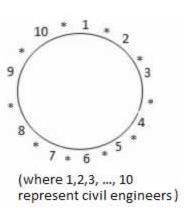This can be done in 10P10 = 10! Ways ---(B)

From (A) and (B),
The required number of ways = 9! × 10!

QUESTION: 19

How many numbers not exceeding 10000 can be made using the digits 2,4,5,6,8 if repetition of digits is allowed?

Solution:

Given that the numbers should not exceed 10000
Hence numbers can be 1 digit numbers or 2 digit numbers or 3 digit numbers
or 4 digit numbers
Given that repetition of the digits is allowed.
A. Count of 1 digit numbers that can be formed using the 5 digits (2,4,5,6,8) (repetition allowed)
The unit digit can be filled by any of the 5 digits (2,4,5,6,8)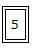Hence the total count of 1 digit numbers that can be formed using the 5 digits (2,4,5,6,8) (repetition allowed) = 5 ---(A)

B. Count of 2 digit numbers that can be formed  using the 5 digits (2,4,5,6,8) (repetition allowed)
Since repetition is allowed, any of the 5 digits(2,4,5,6,8) can be placed
in unit place and tens place.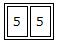Hence the total count of 2 digit numbers that can be formed  using the 5 digits (2,4,5,6,8) (repetition allowed) = 52 ---(B)

C. Count of 3 digit numbers that can be formed using the 5 digits (2,4,5,6,8) (repetition allowed)
Since repetition is allowed, any of the 5 digits (2,4,5,6,8) can be placed  in unit place , tens place and hundreds place.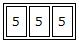Hence the total count of 3 digit numbers that can be formed using the 5 digits (2,4,5,6,8) (repetition allowed) = 53 ---(C)

D. Count of 4 digit numbers that can be formed using the 5 digits (2,4,5,6,8) (repetition allowed)
Since repetition is allowed, any of the 5 digits (2,4,5,6,8) can be placed
in unit place, tens place, hundreds place and thousands place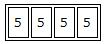Hence the total count of 4 digit numbers that can be formed  using the 5 digits (2,4,5,6,8) (repetition allowed) = 54 ---(D)
From (A), (B), (C), and (D),
total count of numbers not exceeding 10000 that can be made  using the digits 2,4,5,6,8 (with repetition of digits)
= 5 + 52 + 53 + 54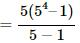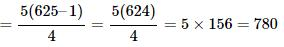QUESTION: 20

In how many ways can 7 identical balls be distributed in 5 different boxes if any box can contain any number of balls?

Solution:

Here n = 5, k = 7. Hence, as per the above formula, the required number of ways